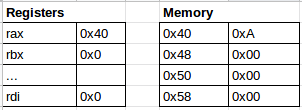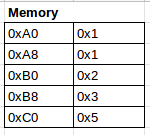In a previous article I showed how to assemble a program using nasm. In this article I’m going to explore different ways to access data and explore some instructions.

## Variables

The simplest way do declare variables is by initializing them in the `.data` segment of a program. The format to define initialized data is:

``````1
[variable-name]    define-directive    initial-value   [,initial-value]...
``````

An example use:

``````1
2
3
4
5
6
7
8
9
10
11
section .data
exit_code dq 0
sys_call dq 60

section .text
global _start

_start:
mov rax, [sys_call]
mov rdi, [exit_code]
syscall
``````

When a variable is defined, some space in memory will be set appart for it. The `dq` directive is used to reserve 64 bits in memory (8 bytes).

Something new in this code snippet is the use of square brackets `[]`. If we didn’t use the brackets, we would be assigning the memory address of the variable instead of the value in that memory address.

If you take a look at the initialization template above, you will notice that you can supply multiple initial values. When this is done, the variable works like an array. i.e. it uses one name to refer to multiple contiguous memory locations:

``````1
some_array dq 1, 1, 2, 3, 5, 8
``````

Something similar can be done for strings, but luckily they allow us to type the whole value instead of having to type one character at a time:

``````1
some_string db "Hello world"
``````

In this case, we used `db` to allocate one byte per character.

To make large strings easier to type, they can be split into multiple lines like this:

``````1
2
some_string db "Hello world, I'm trying to learn assembly, but it's hard. Do "
db "you know what is the fastest way to learn?", 0
``````

The variable name only needs to be specified once, but the `define-directive` needs to be repeated.

## Printing a string

Now that we know how to create strings, let’s try a simple program that prints a string.

Before we start, Let’s look at the interface for syscall `1` (sys_write):

``````1
2
3
rdi   int               file_descriptor
rsi   memory_location   string_to_print
rdx   int               string_size
``````

For `rdi` we will use `1` because that is the file descriptor for stdout. Let’s see how this works in a program:

``````1
2
3
4
5
6
7
8
9
10
11
12
13
14
15
16
17
18
19
section .data
some_string dq "Hello world"
some_string_size dq 11           ; "Hello world" contains 11 characters

section .text
global _start

_start:
; Print the string
mov rax, 1                       ; 1 means sys_write
mov rdi, 1                       ; 1 means stdout
mov rsi, some_string             ; The memory address to the beginning of the string
mov rdx, [some_string_size]      ; Number of characters to print
syscall

; Exit the program
mov rax, 60
mov rdi, 0
syscall
``````

Executing this code will print `Hello world` to the terminal.

## Instructions

Instructions are how we tell the computer to do something. The exact number of instructions on the x64 architecture is hard to find, but it might be somewhere close to one thousand. An instruction consists of an `opcode` and optionally 1 or more `operands`. Let’s look at some common instructions.

### mov

We have already used the `mov` instruction before:

``````1
mov rax, 60
``````

The opcode is `mov` and it receives 2 operands `rax` and `60`. What this instruction does is move the value `60` to the `rax` register.

These are all binary operations. They take two operands and the result will be stored on the first operand:

``````1
2
3
4
mov rax, 60
sub rax, 50    ; rax is now 10
add rax, 5     ; rax is now 15
imul rax, 3    ; rax is now 45
``````

### inc, dec

To increment an operand we can use `inc` and to decrement it, we can use `dec`:

``````1
2
3
mov rax, 60
inc rax      ; rax is 61
dec rax      ; rax is 60 again
``````

### or, xor, and

These are binary bitwise operations:

``````1
2
3
4
mov rax, 5      ; 5 in binary is 101
and rax, 6      ; 6 in binary is 110. rax now holds 4 (100 in binary)
or rax, 8       ; 8 in binary is 1000. rax is now 12 (1100 in binary)
xor rax, 11     ; 11 in binary is 1011. rax is now 7 (111 in binary)
``````

These are just some of the instructions available in an x64 processor. There are many more that I’m not going to cover in this article.

One of the most fundamental things about assembly is understanding addressing modes. An addressing mode is a way to specify which values are going to be used as operands for an instruction. We already used addressing modes in the axamples above. In this section, we are going to give them names and understand them a little more.

### Immediate mode

The immediate mode looks like this:

``````1
mov rax, 60
``````

This mode is very simple because there is no indirection. The `rax` register will be set to `60`. The value `60` is called an immediate constant. Immediate constants can be specified in decimal, binary, octal or hexadecimal. These instructions all do the same:

``````1
2
3
4
mov rax, 60         ; decimal
mov rax, 0b111100   ; binary
mov rax, 0o74       ; octal
``````

### Register mode

This mode is also very easy to understand. Information inside a register will be used:

``````1
mov rax, rbx
``````

In this case, the value of `rax` will be set to whichever value is currently in `rbx`.

### Indirect mode

In this mode, the register contains a memory address, the value we care about, is the value in that memory address:

``````1
mov rdi, [rax]
``````

In the example above, `rax` contains a memory address. `rdi` will be set to the value in that memory address. This is easier to understand with an example. Imagine registers and memory looked like this before executing the instruction above:After the instruction is executed, `rdi` will contain `0xA` because `rax` contains the value `0x40`, which is a memory address. By looking at that memory address, we find the value `0xA`.

We can also use indirect mode for variables, as we did for some of the examples:

``````1
mov rdx, [some_string_size]
``````

With indirect mode, we can also do memory displacements, which is useful for arrays. Assumming we have this array:

``````1
some_array dq 1, 1, 2, 3, 5
``````

We can access its elements like this:

``````1
2
3
4
5
mov rax, [some_array]         ; rax = 1 (first element)
mov rax, [some_array + 8]     ; rax = 1 (second element)
mov rax, [some_array + 16]    ; rax = 2 (third element)
mov rax, [some_array + 24]    ; rax = 3 (fourth element)
mov rax, [some_array + 32]    ; rax = 5 (fifth element)
``````

To understand this a little better we have to remember that each memory address can hold 8 bytes. The `dq` instruction used to create the array, reserves 64 bits per value, so we need 8 addresses to hold a single value (64 / 8 = 8. This is the number of memory addresses it takes to hold a value).

The array looks something like this in memory:Notice that the address after `0xA0` is not `0xA1` but `0xA8`. This is because each number uses 8 memory addresses (64 bits). This way, every displacement on the example above, takes us to the next number in the array.